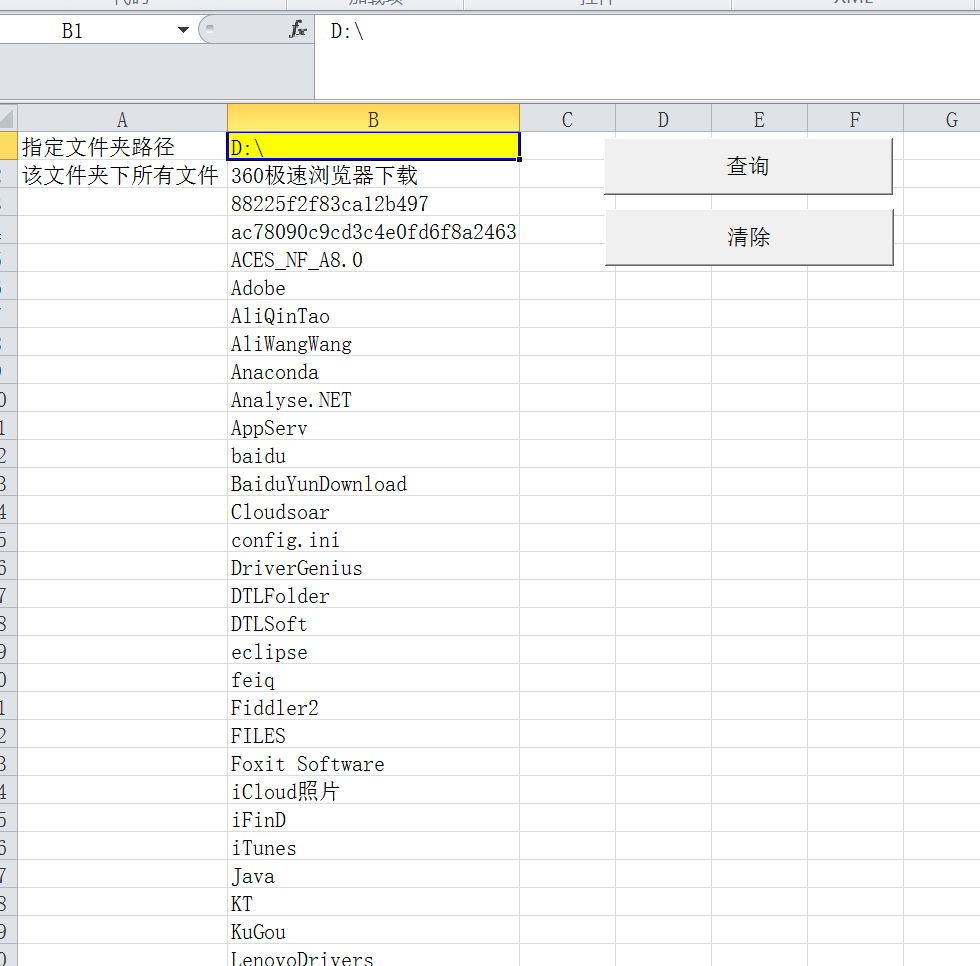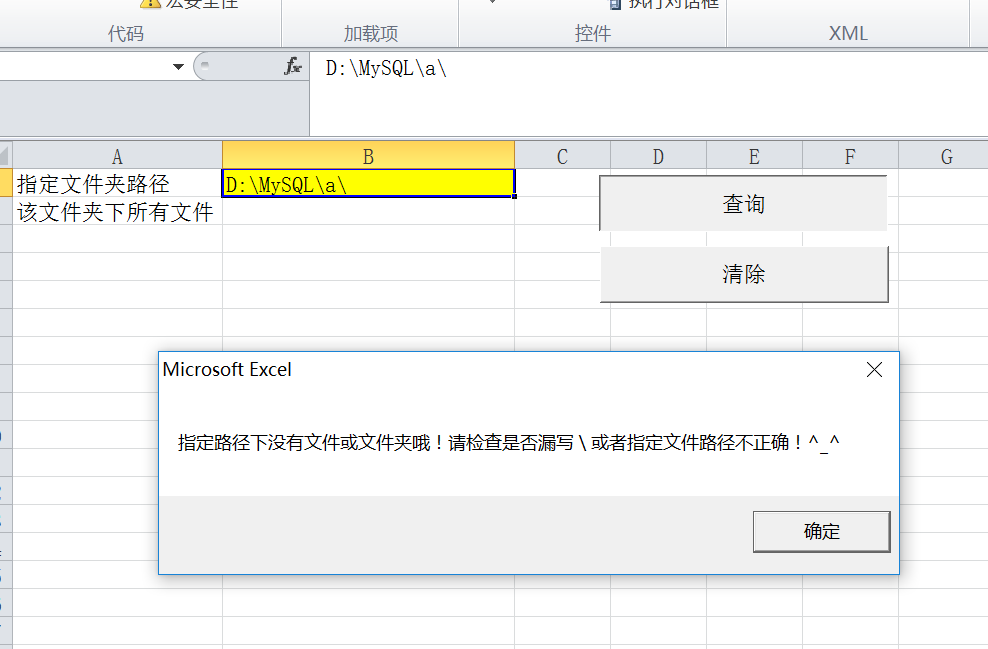【VBA】34. 一个简单的VBA宏（含按钮）

Excel的内容如下所示：Option Explicit

Sub FFInSpecPath(SpecPath As String)
Dim count As Integer
Dim rng As Range
Dim MyFile As String

Set rng = Application.Worksheets("查询文件及文件夹").Range("B2")

MyFile = Dir(SpecPath, vbDirectory)
If MyFile = "" Then
MsgBox ("指定路径下没有文件或文件夹哦！请检查是否漏写 \ 或者指定文件路径不正确！^_^")
End If

Do While MyFile <> ""
MyFile = Dir        '第二次读入的时候不用写参数
If MyFile = "" Then
Exit Do         '当MyFile为空的时候就说明已经遍历完了，这时退出Do，否则还要运行一遍
End If
rng.Offset(count, 0).Value = MyFile
count = count + 1
Loop

End Sub

Sub Call_FFInSpecPath()
Call InitAll
Call FFInSpecPath(Application.Sheets("查询文件及文件夹").Range("B1").Value)
End Sub

Sub InitAll()
Dim lstPath As String
lstPath = Range("B1").Value
Application.Sheets("查询文件及文件夹").Cells.Select
Selection.ClearContents
Range("A1").Value = "指定文件夹路径"
Range("A2").Value = "该文件夹下所有文件"
Range("B1").Value = lstPath
Range("B1").Select

End Sub